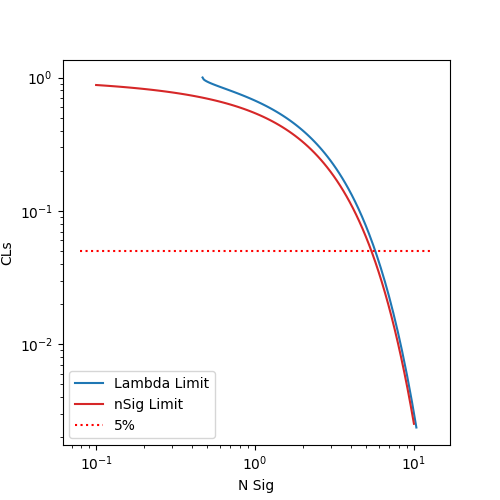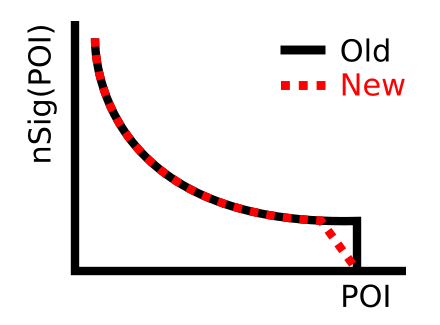Dear Experts,

This is a complicated question, but any insight would be appreciated. I am interested in setting single bin limits on a POI that has a functional dependence on the number of signal events (eg `f(lambda)=nSig`). The problem is that I get a discrepancy between setting limits with `lambda` as the POI and setting limits directly with `nSig` as the POI. For example:

1. Set limits on number of signal events (`nSig`) where `nExp = nSig+nBkg`. For one configuration we get for example an upper limit on `nSig` of <5.40

2. Set limits on `lambda` where a function of `lambda` determines `nSig`: `f(lambda) = nSig`. In this case, `nExp = f(lambda)+nBkg`. With the same configuration we get a limit on lambda that corresponds to `f(lambda)=nSig` of <5.85

It doesn’t seem to be a precision issue since the limits converge on different values when I increase the steps in `SetFixedScan()`. Indeed I get very different CLs values for the two procedures as shown in this plot:I put a stand-alone implementation on Git:

The function `f(lambda)` depends on integrating a number of histograms. The implementation of `f(lambda)` is in a `RooAbsReal` class that simply integrates histograms also saved in the repo. There is a simple script that implements it in analysis/example.py: `cd analysis; python2 example.py`

If anyone has resolved a similar problem, I would be very happy to hear about possible solutions or workarounds.

Best,

Aaron

This topic was automatically closed 14 days after the last reply. New replies are no longer allowed.

Hi @aaronsw,

the first idea that comes up is obviously whether `f(lambda) = nSig`. Can you try to evaluate `f` for some reasonable values of `lambda`, and see if it’s the same (or close to) `nSig`? If that’s not the case, it’s obviously a good explanation for the differences in limits.

The next question is how the sum if sig and bkg are implemented, specifically which class is used to sum the two components. There are some intricacies with AddPdf/RealSumPdf etc, as I tried to summarise in the RooAddPdf documentation:

Hi @StephanH,

Thanks! Aaron Armbruster (not me) was able to help to solve this. I’ll put his explanation here:

When you initialize the b-only model it will do some conditional fits to build the Asimov data, and the small signal there will modify that both through the different fit result you get and through the initial additional signal added to the Asimov. This probably propagates through the rest of the limit calculation.

The change I made is illustrated in this figure. `nSig(POI)` goes smoothly to zero, instead of in a step. Apparently this allows the fit Aaron Armbruster mentions to converge properly.This is the logical conclusion of the check you proposed (checking `f(lambda) = nSig`), so thank you very much for taking a look!

Best,
Aaron

Excellent that you solved this. Thanks a lot for posting the solution!